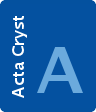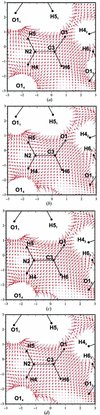## letters to the editorFOUNDATIONSADVANCES
ISSN: 2053-2733
Volume 63| Part 2| March 2007| Pages 201-203

## Response to Spackman's comment on On the calculation of the electrostatic potential, electric field and electric field gradient from the aspherical pseudoatom model

aDepartment of Chemistry, University at Buffalo, State University of New York, Buffalo, NY 14260-3000, USA
*Correspondence e-mail: volkov@chem.buffalo.edu, coppens@buffalo.edu

(Received 3 January 2007; accepted 11 January 2007)

We fully agree with Spackman's tribute to the considerable contribution of R. F. Stewart to the field of charge-density analysis and will restrict our comments to the second half of his letter which discusses a number of issues relevant to the results presented in our paper (Volkov, King et al., 2006).

Mark Spackman (MS) states that it is worth noting that the map presented by VKCF is based on a direct-space summation of contributions from only the eight nearest neighbours in the crystal', implying that the figure presented will change significantly if more neighbouring molecules are included in the calculation. In Fig. 1below, we present analogous maps obtained by taking into account the contributions of pseudoatoms within several unit cells (this option was available in the latest version of XD2006 at the time our original paper was published). Only small changes are observed on the periphery of the map, which do not in any way change any of the conclusions presented in our paper. For the calculation of the electrostatic potential (ESP), electric field (EF) and electric field gradient (EFG), we use the same EPMM approach (Volkov et al., 2004) developed for calculation of the electrostatic interaction energy. Exact formulae are used for atoms located within a certain distance (typically ~6 Å for the ESP, EF and EFG in organic systems) from the point at which the properties are calculated. Beyond that distance, approximate formulae, based on the expansion of the ESP, EF and EFG in atomic multipole moments, are used. Table 1includes a comparison between exact and EPMM methods, demonstrating the satisfactory accuracy of the much faster (Table 2) EPMM method. Table 3shows the convergence of the x and y components of the EF vector in the plane of the map versus the number of unit cells included in the calculation. An excellent convergence is achieved when including atoms with fractional coordinates of −1 < x, y, z < 2, while the inclusion of eight neighbouring cells is also quite satisfactory, i.e. the r.m.s. deviation is only 0.02–0.03 e Å−2, which is less than 1% of the r.m.s. values of the Ex (~5.4 e Å−2) and Ey (~6.5 e Å−2) components of the EF vector in the plane of the map. Note that a similar direct-space approach is used for the calculation of the electrostatic binding energy in the crystal (Volkov et al., 2007), which has also been implemented in XD2006 (Volkov, Macchi et al., 2006). The efficient combination of the exact formulae and the multipole moment approximation eliminates the convergence problems inferred by MS.

 Table 1Root-mean-squared differences in electric field components Ex and Ey (e Å−2) in the plane of the map between exact and EPMM calculations
 (R.m.s. values of Ex and Ey are ~5.4 and ~6.5 e Å−2, respectively.)
8 neighboring molecules All atoms with −1 < x, y, z < 2 All atoms with −2 < x, y, z < 3 All atoms with −3 < x, y, z < 4
R.m.s.(Ex) 2 × 10−6 1 × 10−5 1 × 10−5 1 × 10−5
R.m.s.(Ey) 2 × 10−6 7 × 10−6 1 × 10−5 1 × 10−5
 Table 2Number of atoms and elapsed time (s) for each of the calculations (calculations were performed using Athlon64 3400+ 2.2 GHz processor with 1MB of L2 cache)
Elapsed CPU time (s) Number of atoms used in the
Calculation Exact formulae EPMM calculation
8 neighboring molecules 5.2 4.3 48
All atoms with −1 < x, y, z < 2 54.5 13.6 642
All atoms with −2 < x, y, z < 3 254.5 40.7 2994
All atoms with −3 < x, y, z < 4 713.8 100.8 8226
All atoms with −4 < x, y, z < 5 216.5 17490
 Table 3Root-mean-squared differences in electric field components Ex and Ey (e Å−2) in the plane of the map relative to the values from calculation that included all atoms with −4 < x, y, z < 5 (all from EPMM calculations)
 (R.m.s. values of Ex and Ey are ~5.4 and ~6.5 e Å−2, respectively.)
8 neighboring molecules All atoms with −1 < x, y, z < 2 All atoms with −2 < x, y, z < 3 All atoms with −3 < x, y, z < 4
R.m.s.(Ex) 0.02 0.006 0.0007 0.00020
R.m.s.(Ey) 0.03 0.004 0.0004 0.00009Figure 1 Electric field (EF) vectors in the N2—C3—O1 plane of the central' formamide molecule due to (a) the eight nearest-neighbouring molecules in the crystal, and all atoms with fractional coordinates (b) −1 < x, y, z < 2, (c) −2 < x, y, z < 3 and (d) −4 < x, y, z < 5 (the contribution of the central' molecule to EF is not included). Subscripts of atom names identify the neighbouring molecules. Vectors with magnitudes larger than 0.15 e Å−2 are omitted for clarity. The size of the map is 6 × 6 Å with a grid spacing of 0.2 Å.

MS quotes Cummins et al. (1976) that computation of the EF in the crystal due to point dipoles is well known to require lattice summation techniques to achieve convergence' and adds that these combined approaches are entirely analogous to the Ewald approach used to achieve rapid convergence of lattice sums.' We are of course well aware of the existence of the Ewald summation techniques which we used previously in the calculation of the lattice energy of ionic (Su & Coppens, 1995) and molecular (Abramov, Volkov, Wu & Coppens, 2000a,b; Abramov, Volkov & Coppens, 2000) crystals. However, the direct-space summation was found to be advantageous in terms of both speed and ease of implementation when combined with the new EPMM method (Volkov et al., 2007).

MS states that we misunderstand the purpose of the methods described by Brown & Spackman (1994) as several algorithms published in this paper were used only for debugging of the VALRAY code and should not be considered of practical importance. Nevertheless, the methods were published and are thus in the public domain and subject to further discussion.

The 1957 statement that only the traceless EFG tensor is relevant in the computation of quantities such as nuclear quadrupole coupling constants measured in NMR or NQR experiments (Cohen & Reif, 1957)' is the basis for criticism of our comparison of the elements of the unabridged tensor elements. The point was to verify that our expressions yield correct values, for which the unabridged tensor provides a more stringent test. Going from the total to the traceless definition is of course trivial. In fact XD2006, like Gaussian 03 (2004), automatically prints out both total and traceless components of the EFG tensor as well as the corresponding eigenvalues (Appendix A, Supplementary Material).1 For completeness, the components of the traceless EFG tensor and its eigenvalues for formamide are given in Tables 4and 5. They in no way contradict any of the statements presented in our original paper.

 Table 4Components of the traceless EFG tensor at the nuclear positions in formamide (atomic units) from different methods
XX XY XZ YY YZ ZZ
O(1)
PBE/6-31G** −0.43 −1.52 0.04 1.44 0.02 −1.01
PBE/aug-cc-pVDZ −0.41 −1.50 0.03 1.37 0.02 −0.96
PBE/aug-cc-pVDZ −0.34 −1.49 0.03 1.44 0.02 −1.09
XD/PBE/6-31G** −0.27 −1.21 −0.04 1.25 0.07 −0.98

N(2)
PBE/6-31G** −0.61 −0.03 −0.05 −0.66 −0.01 1.27
PBE/aug-cc-pVDZ −0.58 −0.04 −0.05 −0.63 −0.01 1.20
PBE/aug-cc-pVDZ −0.56 −0.03 −0.05 −0.59 −0.01 1.15
XD/PBE/6-31G** −0.39 −0.02 −0.02 −0.45 −0.02 0.85

C(3)
PBE/6-31G** 0.13 0.08 0.00 0.33 0.00 −0.46
PBE/aug-cc-pVDZ 0.16 0.08 0.00 0.34 0.00 −0.49
PBE/aug-cc-pVDZ 0.19 0.08 0.00 0.39 0.00 −0.59
XD/PBE/6-31G** 0.25 0.07 0.00 0.27 −0.02 −0.52

H(4)
PBE/6-31G** −0.32 −0.42 −0.02 −0.06 −0.01 0.37
PBE/aug-cc-pVDZ −0.31 −0.43 −0.02 −0.05 −0.01 0.37
PBE/aug-cc-pVDZ −0.31 −0.42 −0.02 −0.05 −0.01 0.36
XD/PBE/6-31G** −0.33 −0.44 −0.02 −0.04 −0.01 0.37

H(5)
PBE/6-31G** 0.25 0.07 0.00 −0.60 0.00 0.35
PBE/aug-cc-pVDZ 0.26 0.07 0.00 −0.60 0.00 0.35
PBE/aug-cc-pVDZ 0.25 0.07 0.00 −0.60 0.00 0.34
XD/PBE/6-31G** 0.27 0.07 0.00 −0.62 0.00 0.35

H(6)
PBE/6-31G** 0.21 0.01 0.00 −0.44 −0.01 0.23
PBE/aug-cc-pVDZ 0.22 0.02 0.00 −0.47 −0.01 0.24
PBE/aug-cc-pVDZ 0.20 0.01 0.00 −0.43 −0.01 0.23
XD/PBE/6-31G** 0.21 0.01 0.00 −0.46 0.00 0.24
 Table 5Eigenvalues of the traceless EFG tensor at the nuclear positions in formamide (atomic units) from different methods
λ1 λ2 λ3
O(1)
PBE/6-31G** −1.29 −1.00 2.29
PBE/aug-cc-pVDZ −1.27 −0.95 2.22
PBE/aug-cc-pVDZ −1.20 −1.08 2.28
XD/PBE/6-31G** −0.98 −0.94 1.92

N(2)
PBE/6-31G** −0.68 −0.59 1.27
PBE/aug-cc-pVDZ −0.65 −0.56 1.20
PBE/aug-cc-pVDZ −0.61 −0.54 1.15
XD/PBE/6-31G** −0.46 −0.39 0.85

C(3)
PBE/6-31G** −0.46 0.10 0.35
PBE/aug-cc-pVDZ −0.49 0.13 0.37
PBE/aug-cc-pVDZ −0.59 0.16 0.42
XD/PBE/6-31G** −0.52 0.19 0.33

H(4)
PBE/6-31G** −0.63 0.26 0.37
PBE/aug-cc-pVDZ −0.63 0.26 0.37
PBE/aug-cc-pVDZ −0.63 0.26 0.37
XD/PBE/6-31G** −0.65 0.28 0.37

H(5)
PBE/6-31G** −0.61 0.25 0.35
PBE/aug-cc-pVDZ −0.61 0.26 0.35
PBE/aug-cc-pVDZ −0.60 0.26 0.35
XD/PBE/6-31G** −0.62 0.28 0.35

H(6)
PBE/6-31G** −0.44 0.21 0.23
PBE/aug-cc-pVDZ −0.47 0.22 0.24
PBE/aug-cc-pVDZ −0.43 0.20 0.23
XD/PBE/6-31G** −0.46 0.21 0.24

We agree that use of the Sternheimer correction (Sternheimer, 1986) is questionable and should be re-examined. However, any disagreement between our value and the most precise up-to-date determination of Q(57Fem) (Dufek et al., 1995) is unlikely to be due to the use of the correction, which adds only 0.01 × 10−28 m2 to the uncorrected value of Q(57Fem) = 0.11 × 10−28 m2 (less than 10%). Any disagreement is more likely to be due to experimental errors in the data, which are larger than the Sternheimer correction, as shown in our paper. Of course, this does not eliminate the need for more accurate experimentation and re-examination of the importance of the core polarization.

### Footnotes

1Supplementary material for this paper is available from the IUCr electronic archives (Reference: SH0187 ). Services for accessing this material are described at the back of the journal.

### Acknowledgements

Financial support of this work by the National Science Foundation (CHE0236317) is gratefully acknowledged.

### References

© International Union of Crystallography. Prior permission is not required to reproduce short quotations, tables and figures from this article, provided the original authors and source are cited. For more information, click here.FOUNDATIONSADVANCES
ISSN: 2053-2733
Volume 63| Part 2| March 2007| Pages 201-203
Follow Acta Cryst. A